# 小学四则运算习题程序

GitHub仓库链接（作业存放于学号文件）

PSP2.1 Personal Software Process Stages 预估耗时（分钟） 实际耗时（分钟）
Planning 计划 120 80
Estimate 估计这个任务需要多少时间 120 80
Development 开发 500 600
Analysis 需求分析 (包括学习新技术) 300 240
Design Spec 生成设计文档 30 20
Design Review 设计复审 20 10
Coding Standard 代码规范 (为目前的开发制定合适的规范) 20 10
Design 具体设计 60 55
Coding 具体编码 240 150
Code Review 代码复审 20 15
Test 测试（自我测试，修改代码，提交修改） 40 20
Reporting 报告 40 30
Test Repor 测试报告 10 5
Size Measurement 计算工作量 20 25
Postmortem & Process Improvement Plan 事后总结, 并提出过程改进计划 10 10

## 主要要求：

1.生成的题目中计算过程不能产生负数，也就是说算术表达式中如果存在形如e1−e2的子表达式，那么e1≥e2。
2.生成的题目中如果存在形如e1÷e2的子表达式，那么其结果应是真分数。
3.每道题目中出现的运算符个数不超过3个。
4.程序一次运行生成的题目不能重复，即任何两道题目不能通过有限次交换+和×左右的算术表达式变换为同一道题目。例如，23+45=和45+23=是重复的题目，6×8=和8×6=也是重复的题目。3+(2+1)和1+2+3这两个题目是重复的，由于+是左结合的，1+2+3等价于(1+2)+3，也就是3+(1+2)，也就是3+(2+1)。但是1+2+3和3+2+1是不重复的两道题，因为1+2+3等价于(1+2)+3，而3+2+1等价于(3+2)+1，它们之间不能通过有限次交换变成同一个题目。
6.程序应能支持一万道题目的生成。

## 主要思路:

1.生成表达式：表达式由随机数和运算符两部分组成，开始的时候想的是随机生成数字不就调用一个random函数就搞定的事情，但是到做的时候发现生成符号没有得用了，当时也没想到解决方法，于是查找之后发现可以利用数字来代替这四种计算方式，利用求余的方法得到随机1234四个数
2.随机生成表达式的过程，考虑了之后决定将所有可能的计算情况都列出来，分两种情况，第一种是两个数字一个计算符号，那就是有4种情况，第二种就比较复杂，2个计算符号，那就是4×4=16种情况，要全部列出来其实是比较麻烦，而且代码也会因此变得臃肿，于是我们询问了一下同学，我们发现他们使用的另外一种，就是我们前面使用的随机生成字符的方法。他们是全部生成字符的类型，包括题目的数字，我们感觉这样子后面判断题目答案正误会比较麻烦所以最后还是坚持了我们自己的想法。

## 打码过程：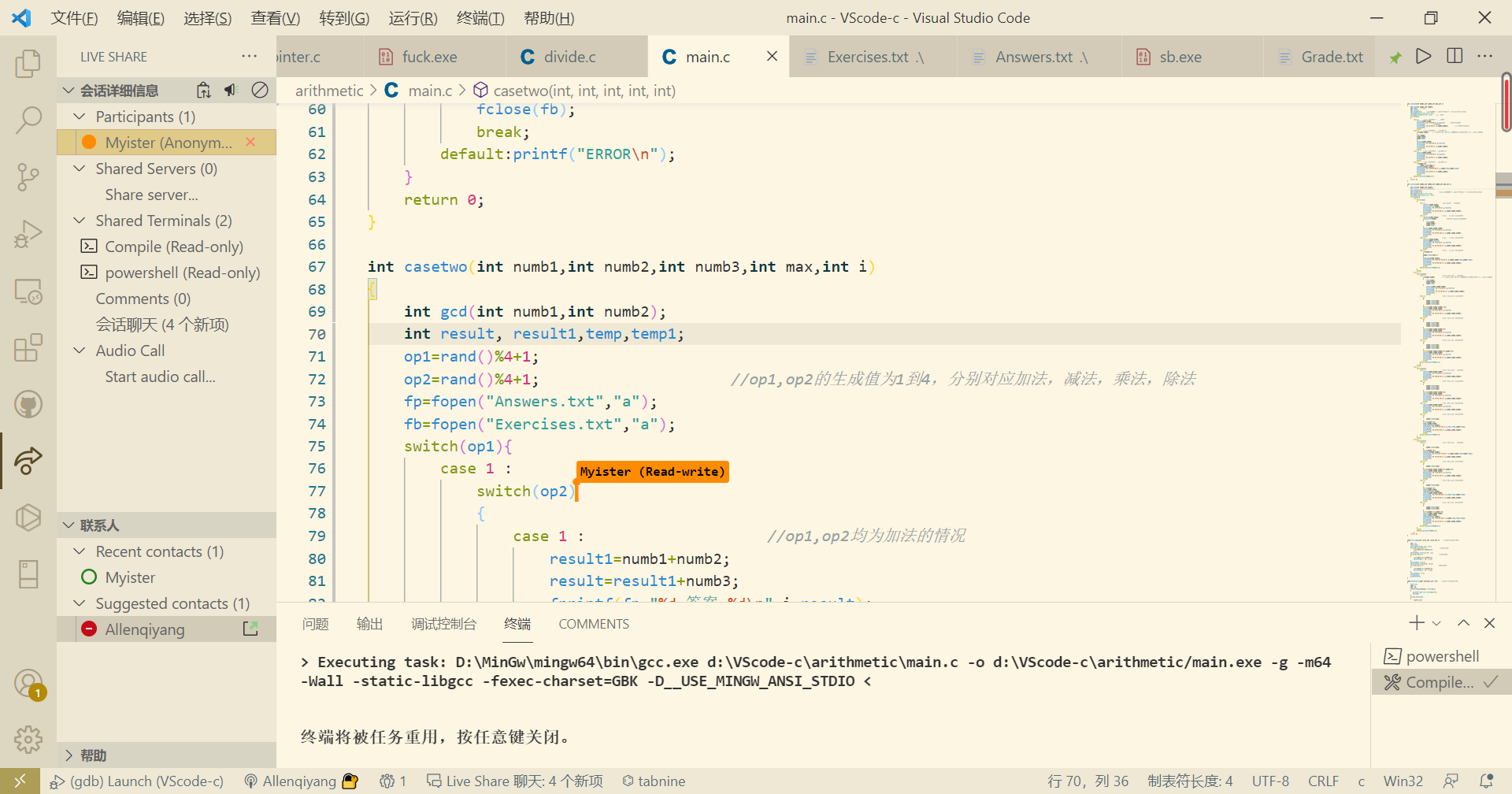## 部分代码


void Put_Judge(int *str1,int *str2,int n)    /* 判断答案对错函数*/
{
int i,j;
FILE *out;
for(i=0,j=0;i<n;i++)                 /*对的个数*/
if(*(str1+i)==*(str2+i))
j++;
fprintf(out,"Correct:%d ",j);
for(i=0;i<n;i++)                    /*对的序号*/
{
if(*(str1+i)==*(str2+i))
fprintf(out," %d ",(i+1));
}
fprintf(out,")\n");
fprintf(out,"Wrong:%d ",(n-j));
for(i=0;i<n;i++)                   /*错的序号*/
{
if(*(str1+i)!=*(str2+i))
fprintf(out," %d ",(i+1));
}
fprintf(out,"\n");
fclose(out);
printf("OK");
}

{
FILE *in;
char a;
int i=0;
if((in=fopen(infile,"r"))==NULL)
{
exit(0);
}
while(!feof(in))
{
*(str+i)=0;
a=fgetc(in);
if(a!='=')
continue;
while(1)
{
a=fgetc(in);
if(a=='\n')break;
*(str+i)*=10;
*(str+i)+=(a-48);
}
printf("%d ",*(str+i));
i++;
}
printf("\n");
fclose(in);
}

int main()
{
int quenum,max;
int opnum;
int caseone(int numb1,int numb2,int max,int i);
int casetwo(int numb1,int numb2,int numb3,int max,int i);
FILE *fp;
fp=fopen("Exercises.txt","w");          //fopen命令w操作当没有文件时会自动建立一个文件
fclose(fp);
fclose(fp);                             //每次打开完一个文件都要及时关闭，否则可能会造成后面出现问题
srand((unsigned)time(0));				//为保证每次运行程序的时候生成的题目都不一样
printf("\t\t随机生成四则运算题目\n");
printf("请输入需要的题目数量n：");
scanf("%d",&quenum);
printf("请输入题目数值范围r：");
scanf("%d",&max);
printf("生成题目\n");
for(i=1;i<=quenum;i++)		        //使用for循环生成题目
{
opnum=rand()%2+1;
switch(opnum)
{
case 1 :							//当随机数是生成1个运算符时
numb1=rand()%max;
numb2=rand()%max;
caseone(numb1,numb2,max,i);		//执行函数的同时的同时执行把题目输出到文件中
break;
case 2 :			                //当随机数是生成2个运算符时
numb1=rand()%max;
numb2=rand()%max;
numb3=rand()%max;
casetwo(numb1,numb2,numb3,max,i);
break;
default:printf("ERROR\n");
}
}
int *exe;
int *ans;
printf("已生成题目，请将Exercises完成\n");
printf("你完成了吗? 完成了请输入1开始批改\n");
scanf("%s",condition);
if(condition == '1')
exe=(int*)malloc(quenum*sizeof(int));
ans=(int*)malloc(quenum*sizeof(int));
Put_Judge(exe,ans,quenum);
return 0;
}


# 效能分析与改进

## 原有的count函数

int Count(char file[])  /*计算的题目数量*/
{
FILE *fp1;
char a;
int x=0;
if((fp1=fopen(file,"r"))==NULL)
{
exit(0);
}
while(!feof(fp1))
{
a=fgetc(fp1);
if(a=='\n')
x++;
}
fclose(fp1);
return x;
}


int main()
{
int subnumber,max;
int opnum;
int caseone(int numb1,int numb2,int max,int i);		//函数的声明
int casetwo(int numb1,int numb2,int numb3,int max,int i);
FILE *fp;
fp=fopen("Exercises.txt","w");
fclose(fp);
fclose(fp);
srand((unsigned)time(0));				//为保证每次运行程序的时候生成的题目都不一样
printf("***************随机生成四则运算题目****************\n");
printf("1.使用 -n 参数控制生成题目的个数\n");
printf("2.使用 -r 参数控制题目中数值的范围\n");
printf("请输入n：");
scanf("%d",&subnumber);
printf("请输入r：");
scanf("%d",&max);
printf("题目生成中，请稍后...\n");
for(i=1;i<=subnumber;i++)		//for循环生成题目
{
opnum=rand()%2+1;
switch(opnum)
{
case 1 :							//当随机数是生成1个运算符时
numb1=rand()%max;
numb2=rand()%max;
caseone(numb1,numb2,max,i);		//执行函数的同时的同时执行把表达式输出到txt文件中
break;
case 2 :			//当随机数是生成2个运算符时
numb1=rand()%max;
numb2=rand()%max;
numb3=rand()%max;
casetwo(numb1,numb2,numb3,max,i);
break;
default:printf("ERROR\n");
}
}
char file1,file2;
int *exe;
int *ans;
printf("请输入练习文本：\n");
scanf("%s",file1);
printf("请输入答案文本：\n");
scanf("%s",file2);
int n;
n=Count(file1);
exe=(int*)malloc(n*sizeof(int));
ans=(int*)malloc(n*sizeof(int));
Put_Judge(exe,ans,n);
return 0;
}


# 实际运行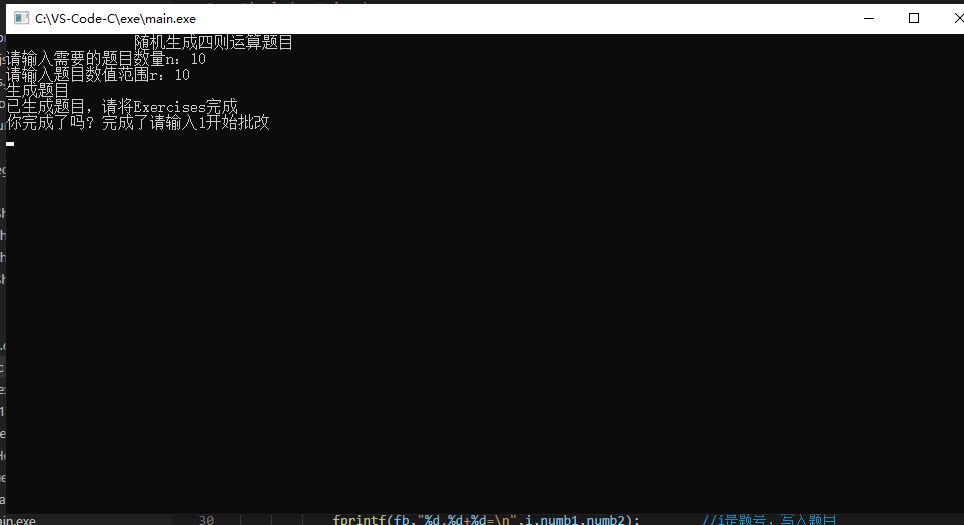## Exercises.txt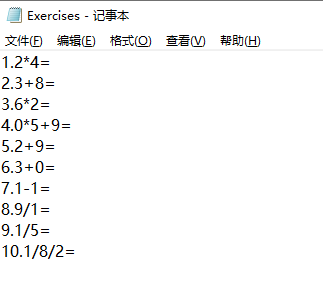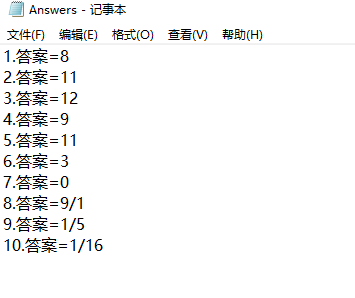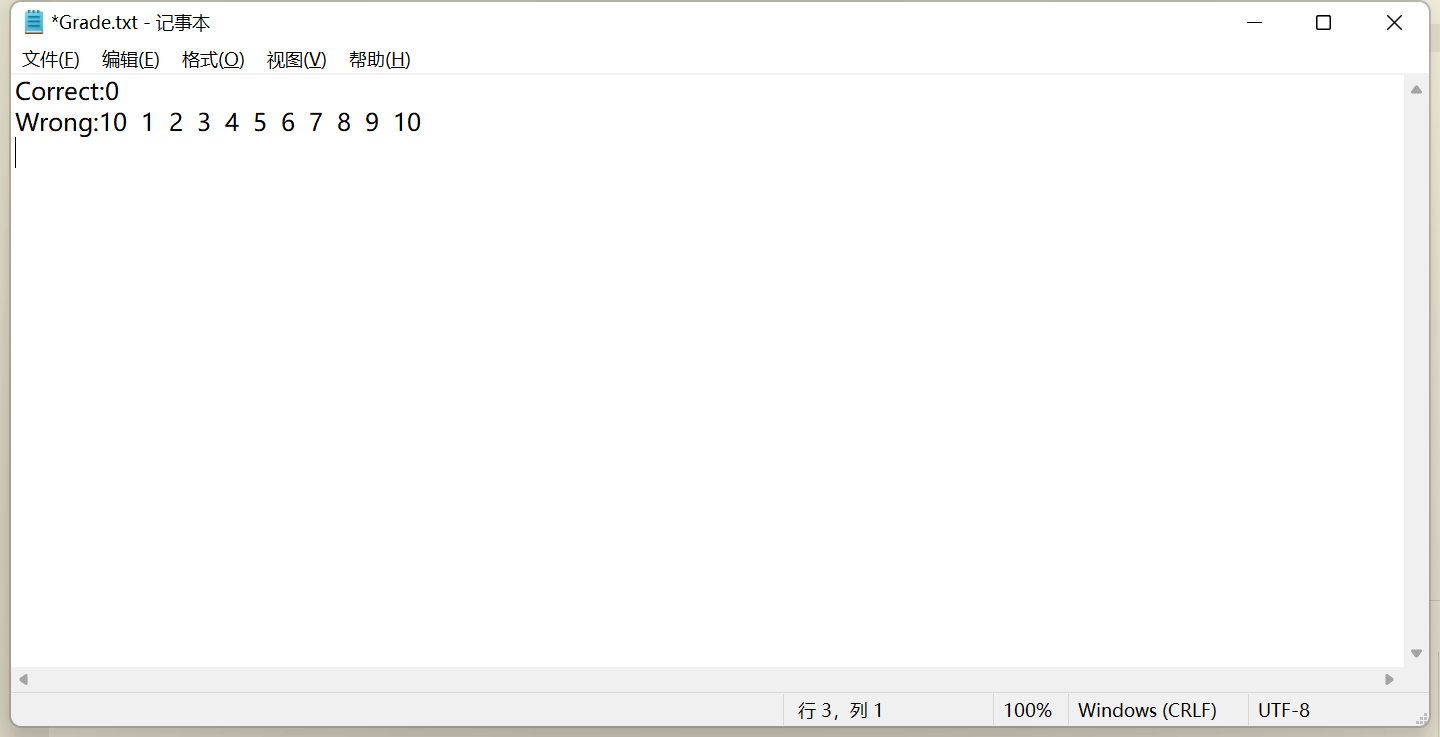## 生成10000道题：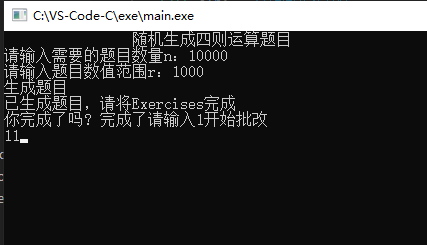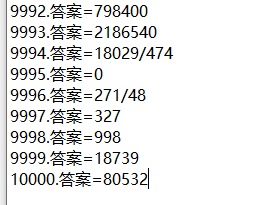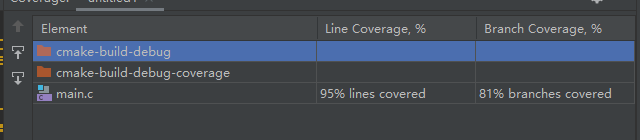## 心得体会：

posted @ 2021-10-10 20:39  lengxing  阅读(126)  评论(0编辑  收藏  举报